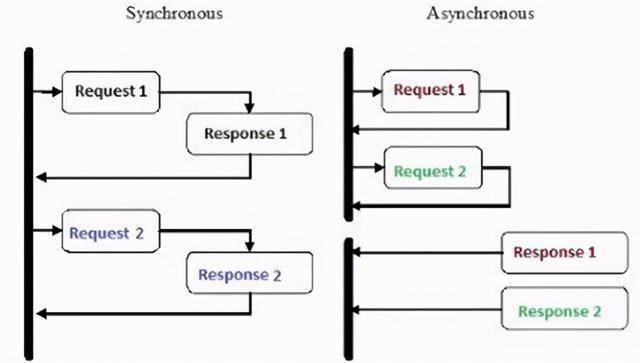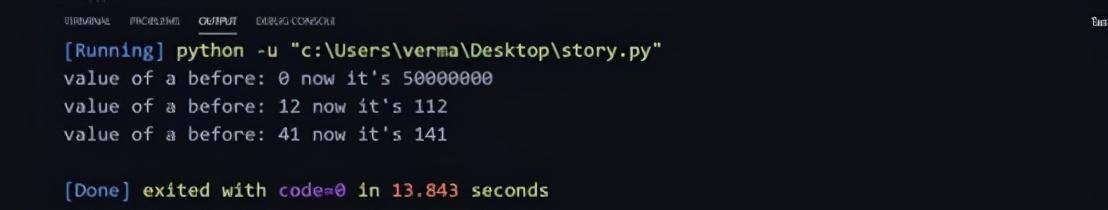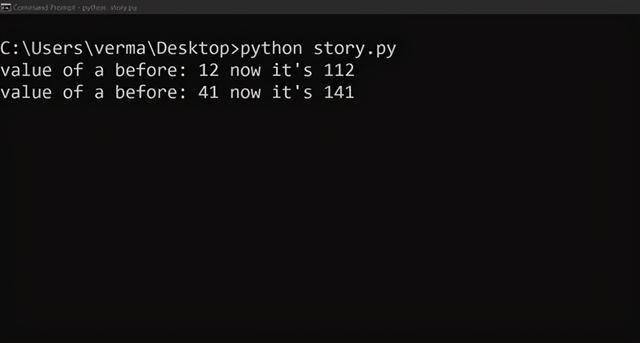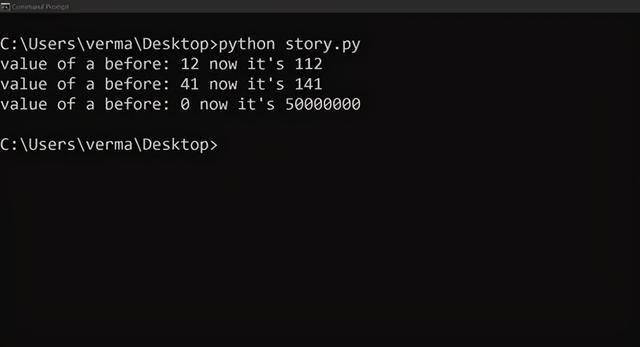### python如何实现异步_在Python中实现异步编程，只需要这几步就够了deffun(length,a): b = a for i inrange(length): a+=1 print(“value of a before: “+str(b)+” now it’s “+str(a)) return a defmain: r1 =fun(50000000,0) r2 =fun(100,12) r3 =fun(100,41) if __name__==”__main__”: mainpip install asyncio

import asyncio asyncdeffun(length,a): b = a for i inrange(length): a+=1 if i %10000==0: await asyncio.sleep(0.0001) print(“value of a before: “+str(b)+” now it’s “+str(a)) return a asyncdefmain: #creating subroutines. t1 = loop.create_task(fun(50000000,0)) t2 = loop.create_task(fun(100,12)) t3 = loop.create_task(fun(100,41)) await asyncio.wait([t1,t2,t3]) if __name__==”__main__”: loop = asyncio.get_event_loop loop.run_until_complete(main) loop.close